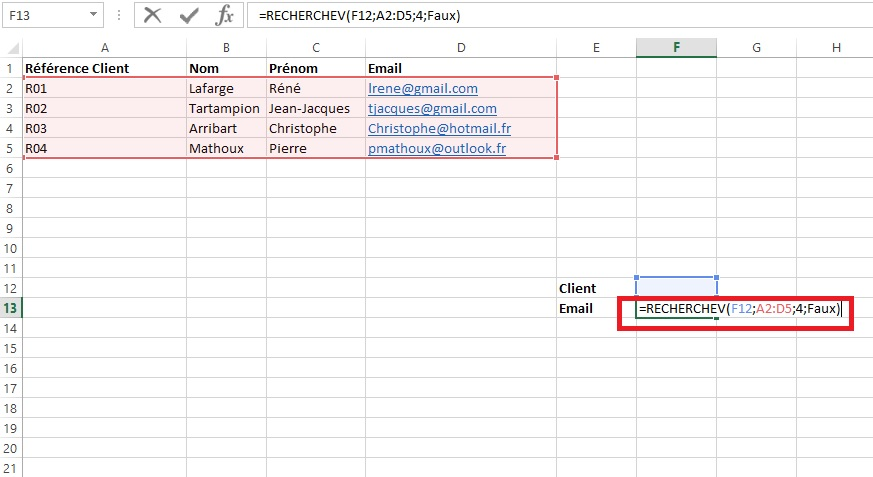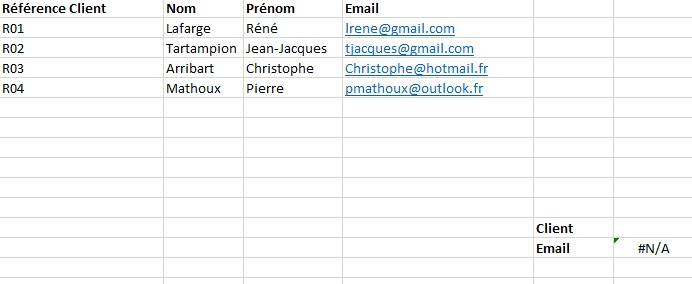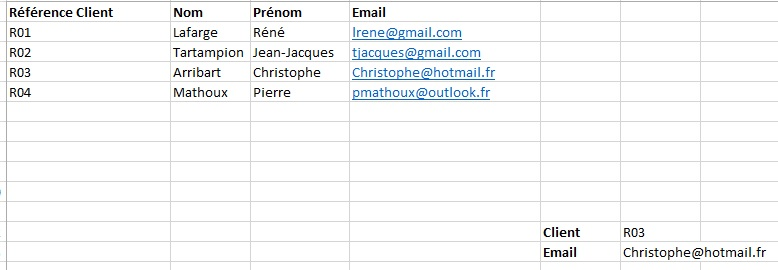# how to use the v search and h search functions

Excel has many features to create useful everyday programs. Whether for business or personal needs, a single spreadsheet can replace an entire application specifically designed for a given task. In this tutorial, we will see how to use the vlookup and hlookup functions in Excel.

Researchv and research are two of the most used functions in Excel. They are used to find data in a dense spreadsheet or even in external spreadsheets. Suppose you have an Excel sheet representing a company’s customer database. Each customer has an identification (reference) and various data associated with his profile.

We want to create a formula to retrieve a customer’s email after having selected his reference in an Excel drop-down list. One such possibility is through the use of the vearch or hearch function.

## Researchv and researchh: how to use them?

Search v and search h in Excel allow, as their name suggests, to retrieve or search for information in a range of data and to return a value corresponding to this reference. An example explained simply is the following: find the customer having for reference R03 in the beach A2 to D5 and retrieves his email address. We will see how to translate this query into a formula in Excel.

But before coming to that, let us specify that the difference between researchv and researchh is at the level of the direction of the research. V for vertical (column) and H for horizontal (row). Here’s how to use them in a formula:

1. Searchv (lookup_value; array; column; near_value)
2. H lookup (lookup_value; array; row; near_value)
• Desired value : it is the value which must be sought in the range or matrix in order to find a specific data concerning it
• Matrix : this is the range of cells in which the value to search is located
• Column or row number : this parameter corresponds to the number of the column (vrearch) or row (hrearch) which contains the expected result. It is necessary to count from the first column of the selected range and not from the first column of the worksheet
• Close value : this is a logical (or boolean) argument that can only have two states: true or false. It is used to indicate whether one wishes to recover a result with a close value or an exact value corresponding to the requested_value. Very often, we are looking for a result that exactly matches the value we are looking for. You will need to enter the False argument for this.

Read also: How to round up or down in Excel

## Practical case

Finally, here is a concrete example that will allow you to better understand all these explanations. We are going to create a formula to get the email address of a customer by entering his reference in a cell. To do this, we will use the vearch formula since the references are listed one after the other vertically.As you can see from the screenshot above, the formula looks like this: = VLOOKUP (F12; A2: D5; 4; FALSE). We have the database (range of cells) on the one hand. It covers the first 5 rows of columns A to D.

Further down, in columns E and F, we have created two rows to go find a value and return the corresponding result. In this case, cell F12 must contain the value to search for (customer reference). Cell F13 contains the formula for fetching the customer’s email.

• The first argument of our formula is therefore F12 since we will look for the reference entered in this cell
• The second argument is A2: D5 which corresponds to the range or matrix which contains both the reference and the sought value
• The third argument is the number 4 which corresponds to the index of the column that contains the search result. In this case, the emails are in column D which is the 4th column starting from the first column of the selected range
• Finally, the FALSE argument allows you to find the exact match. It makes no difference here since it is a string.

By validating the formula, the cell displays an N / A error which is quite normal. To display a value, you must enter the reference of a customer in cell F12 to retrieve his email which will therefore be displayed in the cell containing the search formula (F13).This is not very complicated. The operation is practically the same for the Searchh function which should be used if the list of the search source is arranged horizontally, which is not the case in our example.